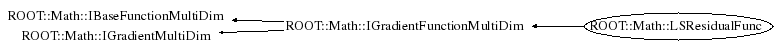```
LSResidualFunc class description.
Internal class used for accessing the residuals of the Least Square function
and their derivates which are estimated numerically using GSL numerical derivation.
The class contains a pointer to the fit method function and an index specifying
the i-th residual and wraps it in a multi-dim gradient function interface
The class is used by ROOT::Math::GSLNLSMinimizer (GSL non linear least square fitter)

@ingroup MultiMin

```

## Function Members (Methods)

public:
 ~LSResidualFunc() virtual ROOT::Math::IMultiGenFunction* Clone() const double ROOT::Math::IGradientMultiDim::Derivative(const double* x, unsigned int icoord = 0) const virtual void ROOT::Math::IGradientFunctionMultiDim::FdF(const double* x, double& f, double* df) const virtual void Gradient(const double* x, double* g) const ROOT::Math::LSResidualFunc LSResidualFunc() ROOT::Math::LSResidualFunc LSResidualFunc(const ROOT::Math::LSResidualFunc& rhs) ROOT::Math::LSResidualFunc LSResidualFunc(const ROOT::Math::FitMethodFunction& func, unsigned int i) virtual unsigned int NDim() const double ROOT::Math::IBaseFunctionMultiDim::operator()(const double* x) const ROOT::Math::LSResidualFunc& operator=(const ROOT::Math::LSResidualFunc& rhs)
private:
 virtual double DoDerivative(const double* x, unsigned int icoord) const virtual double DoEval(const double* x) const

## Data Members

private:
 const ROOT::Math::FitMethodFunction* fChi2 unsigned int fIndex vector fX2 cached vector

## Class Charts## Function documentation

LSResidualFunc(const ROOT::Math::LSResidualFunc& rhs)
```default ctor (required by CINT)
```
`{}`
LSResidualFunc(const ROOT::Math::FitMethodFunction& func, unsigned int i)
`{}`
LSResidualFunc(const ROOT::Math::LSResidualFunc& rhs)
``` copy ctor
```
operator=(const ROOT::Math::LSResidualFunc& rhs)
IMultiGenFunction * Clone()
unsigned int NDim()
`{ return fChi2->NDim(); }`
void Gradient(const double* x, double* g) const
double DoEval(const double* x) const
double DoDerivative(const double* x, unsigned int icoord) const
```return  ROOT::Math::Derivator::Eval(*this, x, icoord, 1E-8);
```

Author: L. Moneta Wed Dec 20 17:16:32 2006
Last update: root/mathmore:\$Id: GSLNLSMinimizer.h 21553 2007-12-21 10:55:46Z moneta \$
Copyright (c) 2006 LCG ROOT Math Team, CERN/PH-SFT *

This page has been automatically generated. If you have any comments or suggestions about the page layout send a mail to ROOT support, or contact the developers with any questions or problems regarding ROOT.